### Finite Element Analysis: FEA in Two and Three Dimensions

two-dimensional elements is presented.

### Stiffness Matrix and Nodal Forces Vector for a General 3D Linear Elastic Element

In three dimensions, the displacement vector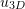of an element has three components designated,, andsuch that: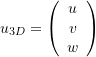Assuming that the element hasnodes, then, each nodehas 3 nodal degrees of freedom designated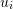,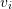, and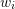. So, the nodal degrees of freedom vector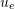can have the form: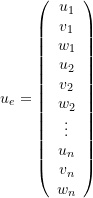If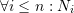is the shape function associated with node, then the displacement vector function has the form: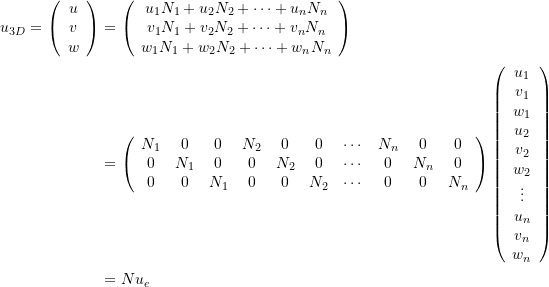For simplicity, the vector representation of the small strain matrix will be used. In this case, the small strain matrix can be written in vector form as a function of the nodal degrees of freedom vector as follows: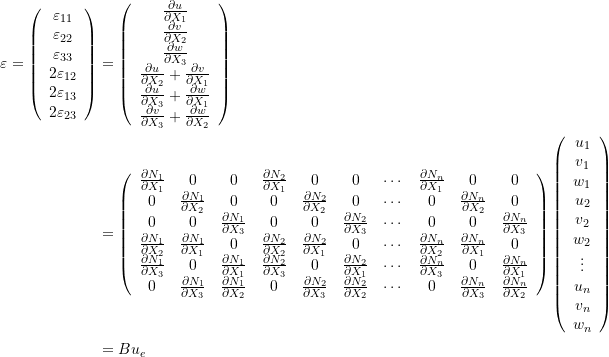For a general linear elastic material, the vector representation of the stress matrix is related to the vector representation of the small strain matrix by a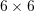symmetric material coefficients matrix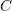: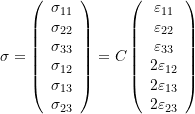Utilizing the principle of virtual work, the virtual displacement field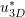and the associated virtual strain field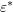can have the following forms with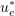being an arbitrary “virtual” vector of degrees of freedom of the system: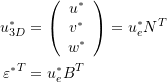where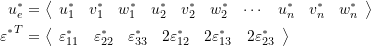The internal virtual work integral term for this particular element will have the form:where the integration is done on each term in the matrix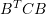and is performed over the volume (in 3D problems) of the element. The local stiffness matrix has dimensions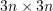and has the form: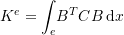The external virtual work integral term for this particular element will have the form: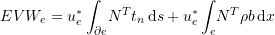Where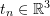is the traction vectors on the surface of the element,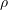is the mass density, and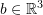is the body forces vector on the element. The integration in the first term is done over the element surface while in the second term is done over the element volume. The local nodal forces vector will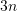components and will have the form: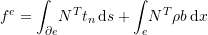### Stiffness Matrix and Nodal Forces Vector for a General 2D Linear Elastic Element

The equations in the previous section are repeated after reducing them to two dimensions. In this case, the displacement vector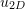of an element has two components designatedandsuch that: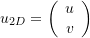Assuming that the element hasnodes, then, each nodehas 2 nodal degrees of freedom designatedand. So, the nodal degrees of freedom vectorcan have the form: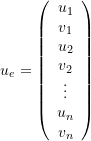Ifis the shape function associated with node, then the displacement vector function has the form:For simplicity, the vector representation of the small strain matrix will be used. In this case, the small strain matrix can be written in vector form as a function of the nodal degrees of freedom vector as follows: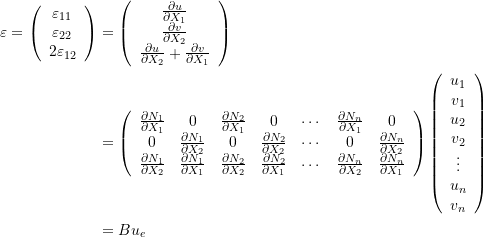For a general plane strain or plane stress linear elastic material, the vector representation of the stress matrix is related to the vector representation of the small strain matrix by a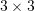symmetric material coefficients matrix: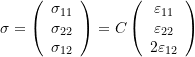Utilizing the principle of virtual work, the virtual displacement field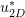and the associated virtual strain fieldcan have the following forms withbeing an arbitrary “virtual” vector of degrees of freedom of the system: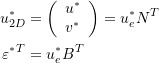where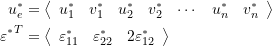The internal virtual work integral term for this particular element will have the form:where the integration is done on each term in the matrixand is performed over the volume (which in the 2D case would be an integration over the area multiplied by the thickness) of the element. The local stiffness matrix has dimensions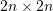and has the form:The external virtual work integral term for this particular element will have the form:Where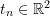is the traction vectors on the surface of the element,is the mass density, and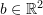is the body forces vector on the element. The integration in the first term is done over the element surface while in the second term is done over the element volume. The local nodal forces vector will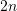components and will have the form: